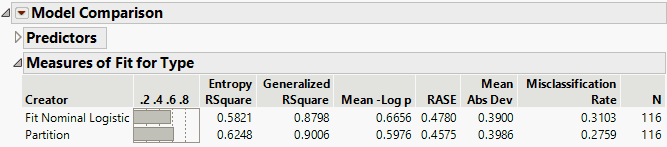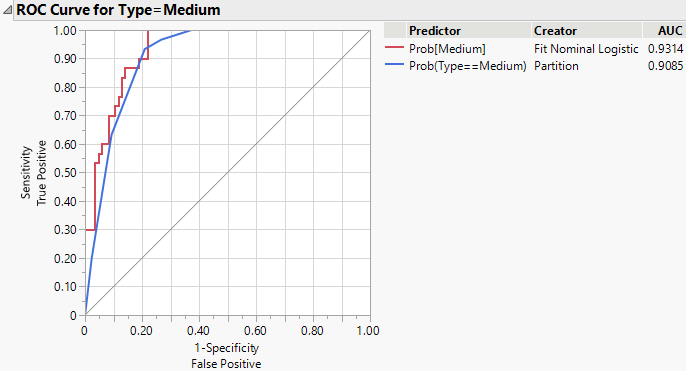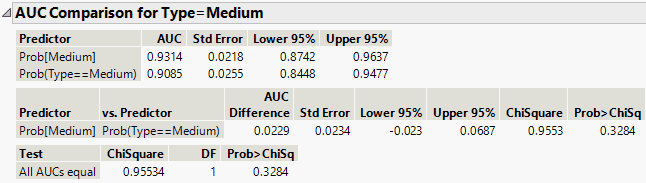Predictive and Specialized Modeling > Model Comparison > Additional Example of Model Comparison
Publication date: 05/24/2021

#Additional Example of Model Comparison

This example uses automobile data to build a model to predict the size of the purchased car. A logistic regression model and a decision tree model are compared.

Begin by selecting Help > Sample Data Library and opening Car Physical Data.jmp.

#### Create the Logistic Regression Model

1. Select Analyze > Fit Model.

2. Select Type and click Y.

3. Select the following columns and click Add: Country, Weight, Turning Cycle, Displacement, and Horsepower.

4. Click Run.

The Nominal Logistic Fit report appears.

5. To save the prediction formulas to columns, click the Nominal Logistic red triangle and select Save Probability Formula.

#### Create the Decision Tree Model and Save the Prediction Formula to a Column

1. Select Analyze > Predictive Modeling > Partition.

2. Select Type and click Y, Response.

3. Select the Country, Weight, Turning Cycle, Displacement, and Horsepower columns and click X, Factor.

4. Make sure that Decision Tree is selected in the Method list.

5. Click OK.

The Partition report appears.

6. Click Split 10 times.

7. To save the prediction formulas to columns, click the Partition red triangle and select Save Columns > Save Prediction Formula.

#### Compare the Models

1. Select Analyze > Predictive Modeling > Model Comparison.

2. Select all columns that begin with Prob and click Y, Predictors.

3. Click OK.

Figure 11.8 Initial Model Comparison ReportThe report shows that the Partition model has slightly higher values for Entropy RSquare and Generalized RSquare and a slightly lower value for Misclassification Rate.

4. Click the Model Comparison red triangle and select ROC Curve.

ROC curves appear for each Type, one of which is shown in Figure 11.9.

Figure 11.9 ROC Curve for MediumExamining all the ROC curves, you see that the two models are similar in their predictive ability.

5. Click the Model Comparison red triangle and select AUC Comparison.

AUC Comparison reports appear for each Type, one of which is shown in Figure 11.10.

Figure 11.10 AUC Comparison for MediumThe report shows results for a hypothesis test for the difference between the AUC values (area under the ROC curve). Examining the results, you see there is no statistical difference between the values for any level of Type.

You conclude that there is no large difference between the predictive abilities of the two models for the following reasons:

The R Square values and the ROC curves are similar.

There is no statistically significant difference between AUC values.